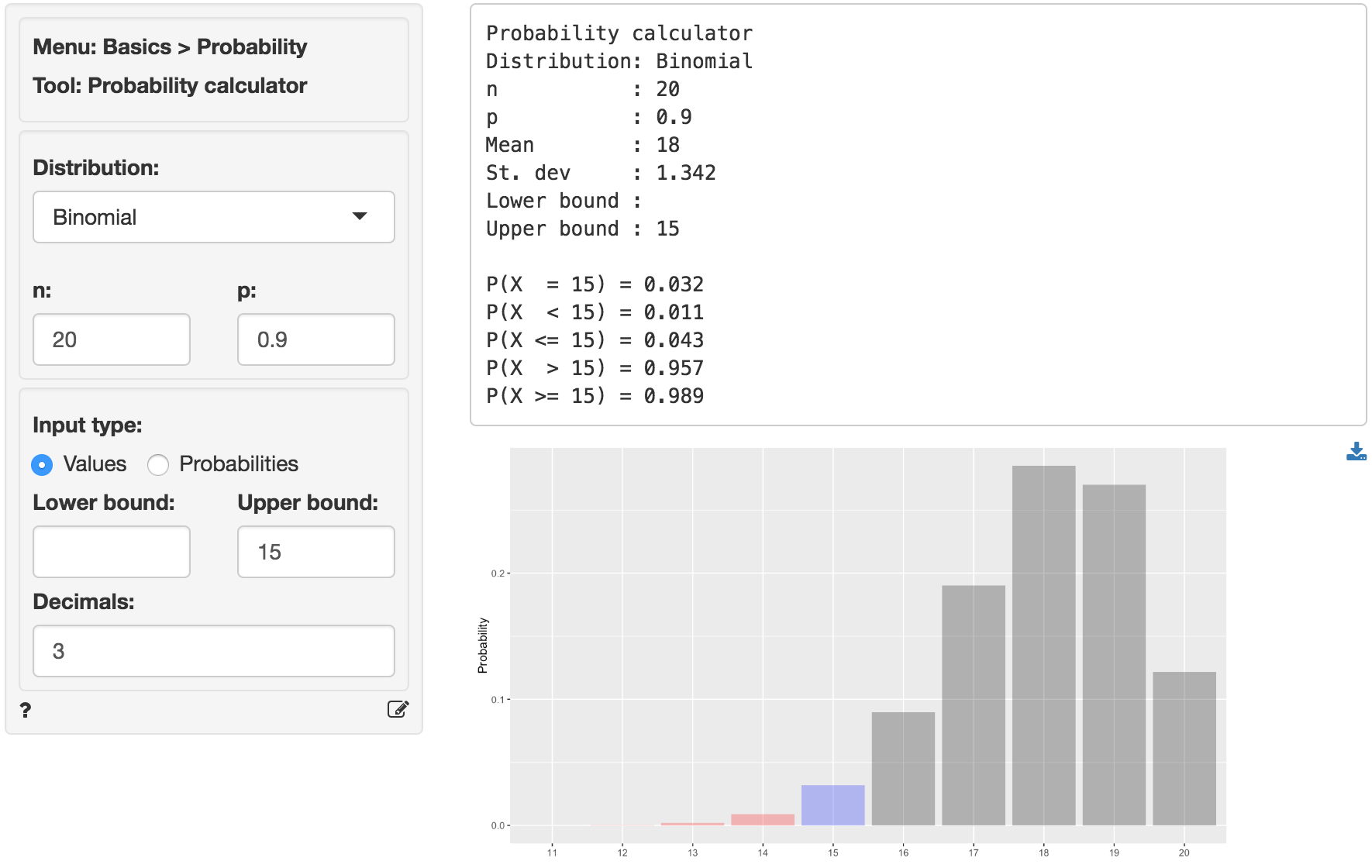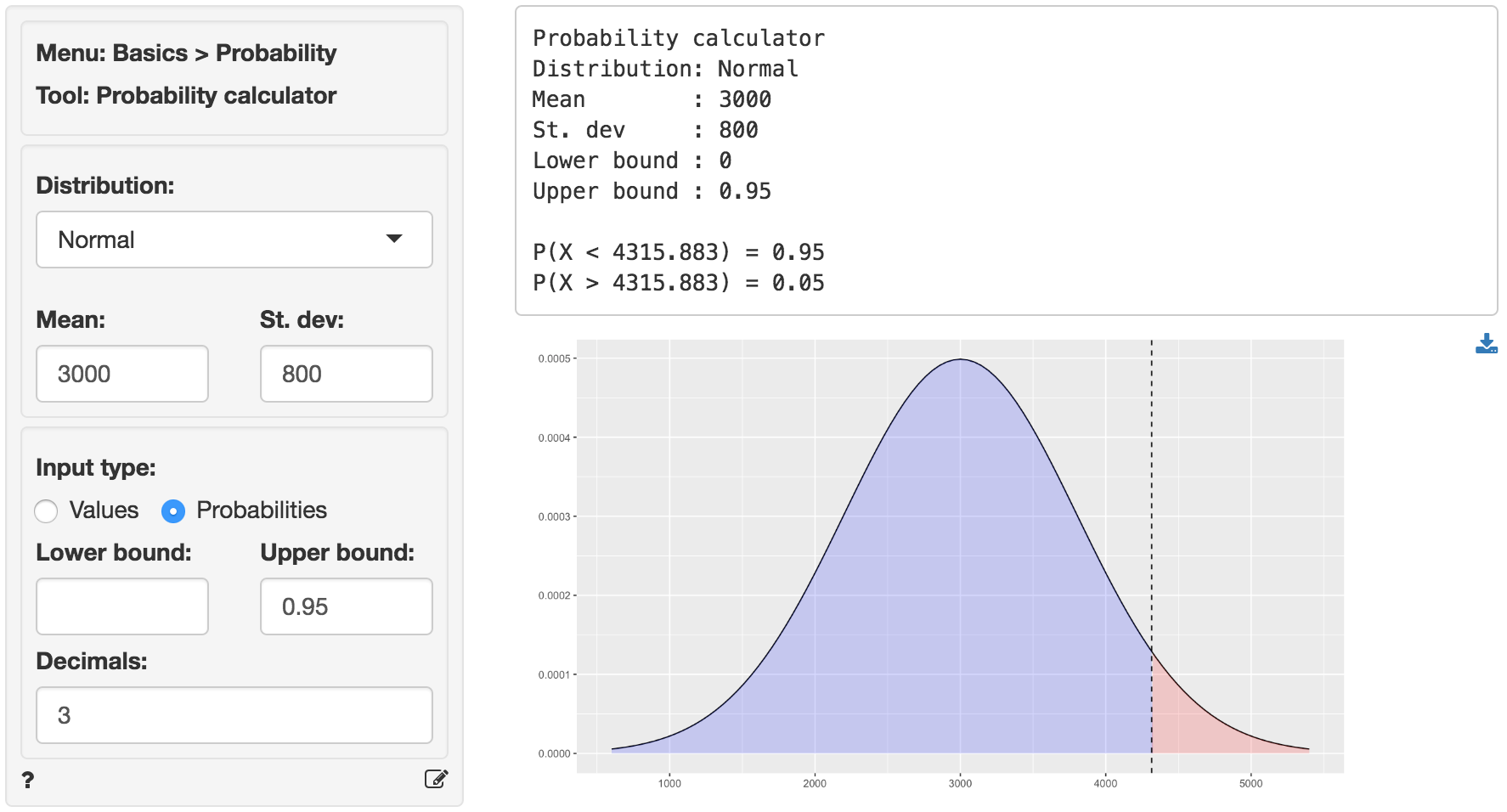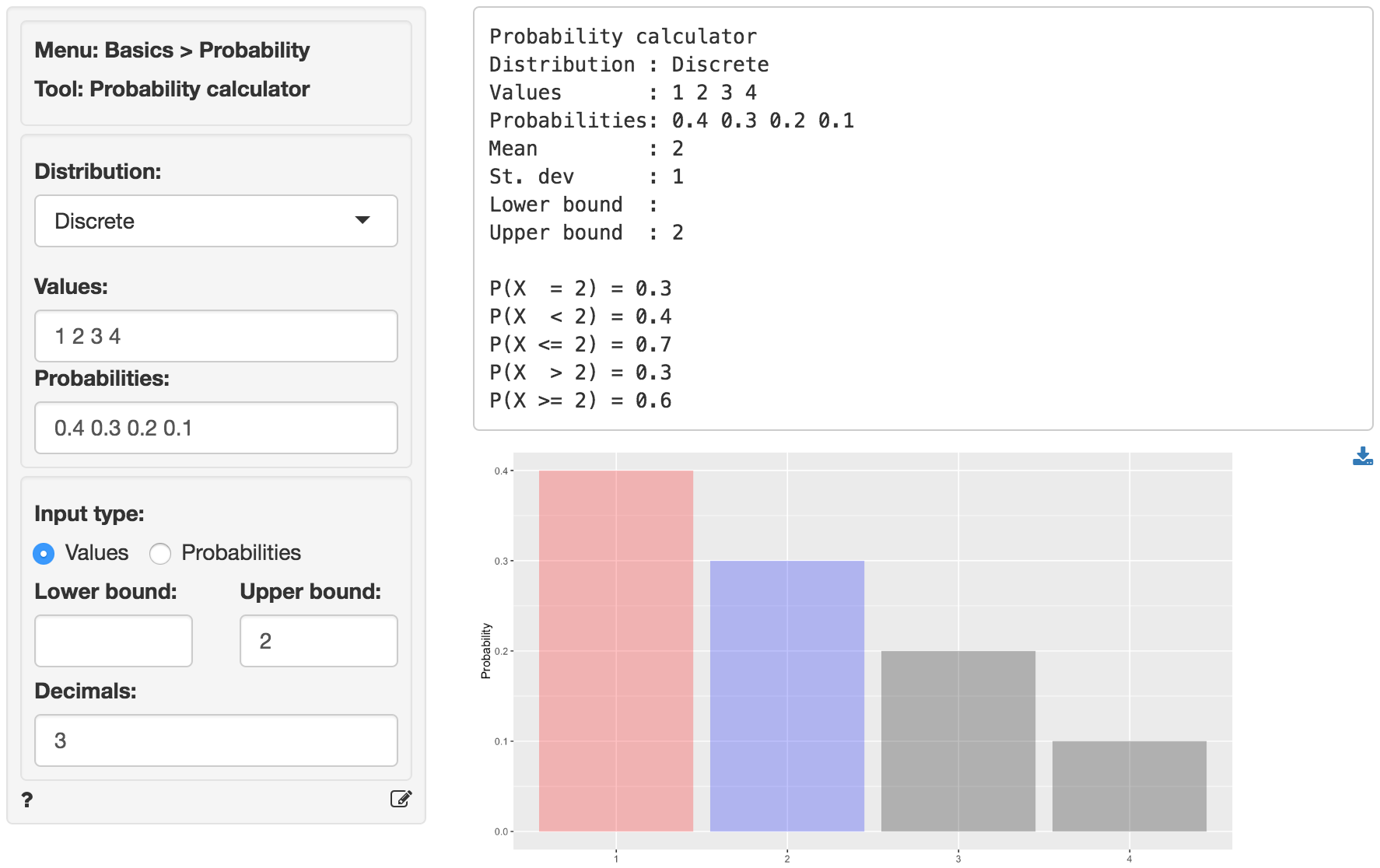Probability calculator

Calculate probabilities or values based on the Binomial, Chi-squared, Discrete, F, Exponential, Normal, Poisson, t, or Uniform distribution.

## Testing batteries

Suppose Consumer Reports (CR) wants to test manufacturer claims about battery life. The manufacturer claims that more than 90% of their batteries will power a laptop for at least 12 hours of continuos use. CR sets up 20 identical laptops with the manufacturer’s batteries. If the manufacturer’s claims are accurate, what is the probability that 15 or more laptops are still running after 12 hours?

The description of the problem suggests we should select Binomial from the Distribution dropdown. To find the probability, select Values as the Input type and enter 15 as the Upper bound. In the output below we can see that the probability is 0.989. The probability that exactly 15 laptops are still running after 12 hours is 0.032.A manufacturer wants to determine the appropriate inventory level for headphones required to achieve a 95% service level. Demand for the headphones obeys a normal distribution with a mean of 3000 and a standard deviation of 800.

To find the required number of headphones to hold in inventory choose Normal from the Distribution dropdown and then select Probability as the Input type. Enter .95 as the Upper bound. In the output below we see the number of units to stock is 4316.## Cups of ice cream

A discrete random variable can take on a limited (finite) number of possible values. The probability distribution of a discrete random variable lists these values and their probabilities. For example, probability distribution of the number of cups of ice cream a customer buys could be described as follows:

• 40% of customers buy 1 cup;
• 30% of customers buy 2 cups;
• 20% of customers buy 3 cups;
• 10% of customers buy 4 cups.

We can use the probability distribution of a random variable to calculate its mean (or expected value) as follows;

$E(C) = \mu_C = 1 \times 0.40 + 2 \times 0.30 + 3 \times 0.20 + 4 \times 0.10 = 2\,,$

where $$\mu_C$$ is the mean number of cups purchased. We can expect a randomly selected customer to buy 2 cups. The variance is calculated as follow:

$Var(C) = (1 - 2)^2 \times 0.4 + (2 - 2)^2 \times 0.3 + (3 - 2)^2 \times 0.2 + (4 - 2)^2 \times 0.1 = 1\,.$

To get the mean and standard deviation of the discrete probability distribution above, as well as the probability a customer will buy 2 or more cups (0.6), specify the following in the probability calculator.## Hypothesis testing

You can also use the probability calculator to determine a p.value or a critical value for a statistical test. See the helpfiles for Single mean, Single proportion, Compare means, Compare proportions, Cross-tabs in the Basics menu and Linear regression (OLS) in the Model menu for details.

© Vincent Nijs (2016)